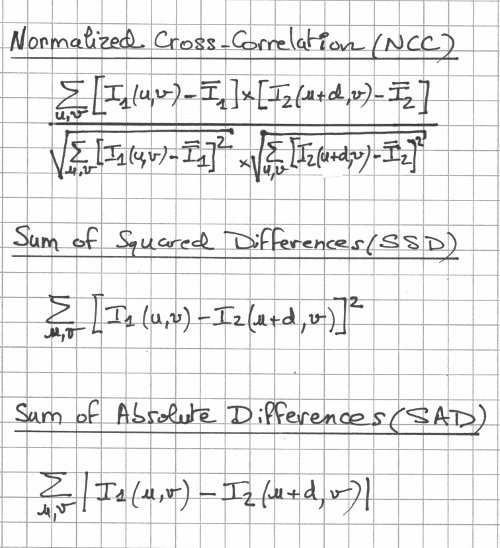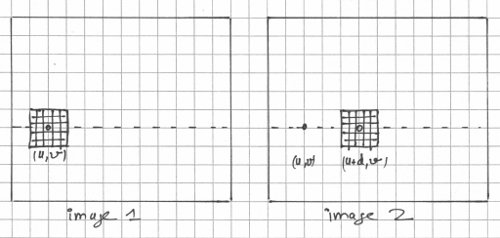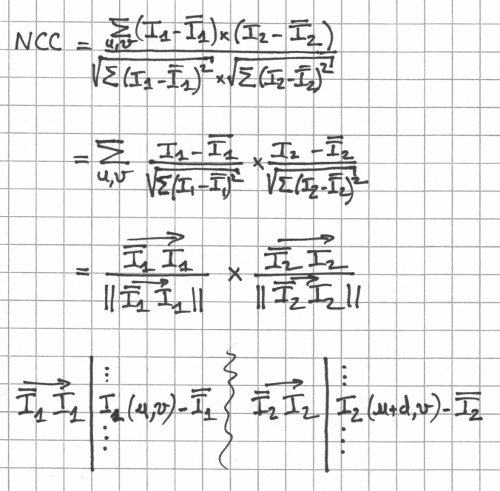## Monday, January 2, 2012

### Stereo Matching - Local Methods

In stereo matching (correspondence), local methods attempt to match two dimensional windows (blocks) on the left and right images using a winner-take-all approach (best match wins). They vary by how they compute the matching cost (what matching metric is used) and how they aggregate the cost (how far around the pixel of interest they go). They are local not because of the way they compute the cost but because of the way the problem is solved: For each pixel on the left or right image, a matching pixel is found on the "target" image independently of the other pixels. Contrast this with global methods which minimize the energy of the whole system. Local methods are (much) faster than global methods, that's why they are quite popular. The depth maps obtained by using local matching methods typically suffer from a lack of smoothness, and that's where the global methods come in (we'll check those out in another post).

Let's have a look at the most popular matching metrics in no particular order:Normalized Cross-Correlation (NCC), Sum of Squared Differences (SSD), Sum of Absolute Differences (SAD) matching metrics.

Most stereo matching algorithms do not make use of RGB color information as they only consider the intensity I, that is, the gray scale value (which varies from 0 to 255). In the formulas, I_bar is the mean intensity value and d is the disparity. The summation is over a window which is usually but not necessarily centered on the pixel to match. The formulas assume that the matches are made along a scan line (v).

Matching a pixel from image 1 to image 2 requires the computation of the matching cost with the disparity d varying from its minimum value to its maximum value (usually given). The lowest cost is taken as the winner (winner-takes-all) and a match is made. It's kinda like sliding (pixel by pixel) the window along the scan line in image 2 and picking the best match (lowest matching cost). Maybe a picture might help:Window-based stereo matching.

The normalization process in Normalized Cross-Correlation (NCC) reduces the effect of intensity variations between the two images by subtracting the mean from the intensity. Dividing by the standard deviations restricts the Normalized Cross-Correlation to the range [-1,1]. The physical meaning of Normalized Cross Correlation may possibly be better understood if it is shown to be the dot product of two normalized vectors of dimension w x h (where w and h define the window width and height, respectively).Normalized Cross Correlation (NCC) as the dot product of 2 normalized vectors.

There is no ideal window size: must be big enough to have enough intensity variation to ensure proper matching, but small enough to avoid the effects of perspective distortion (how an object looks against a background usually depends on the point of view).

There are other matching metrics but these three (NCC, SSD, and SAD) are the most common. Which one is the best? It kinda depends on who you are talking to, the kind of images you are dealing with, and how fast you want the matches to be made (clearly, NCC is slower than SSD or SAD).